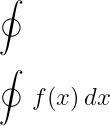# How do I type a closed integral(∮) in Latex?

Close integrals are represented by adding circles to the integral symbols. And lower limit and upper are not given while representing Close Integral. Latex has more than one command to denote this mathematical symbol.

And for a close integral symbol, you should always use an external package.

## Use esint package for close integral symbol

You can use \oint command to represent closed integral symbols in esint packages.

\documentclass{article}
\usepackage{esint}
\begin{document}
$$\oint$$
$$\oint\, f(x)\, dx$$
\end{document}

Output :You probably know about Surface Closed Integral. Mathematically, the closed double integral symbol is used to represent the surface closed integral.

In this case, you can use both \oiint and \varoiint commands.

\documentclass{article}
\usepackage{esint}
\begin{document}
$$\varoiint_{s} f(x,y)dxdy$$
$$\oiint_{s} f(y,z)dydz$$
\end{document}

Output :\varoiint command is only used to represent a closed surface integral symbol.

## Use wasysym package for close integral symbol

\oint and \oiint commands are present in wasysym package to represent closed line and surface Integral without esint package.

\documentclass{article}
\usepackage{wasysym}
\begin{document}
$$\oint_{\theta}F(\theta)d{\theta}$$
$$\oiint_{s}F(x,z)dxdz$$
\end{document}

Output :#### Md Jidan Mondal

LaTeX expert with over 10 years of experience in document preparation and typesetting. Specializes in creating professional documents, reports, and presentations using LaTeX.